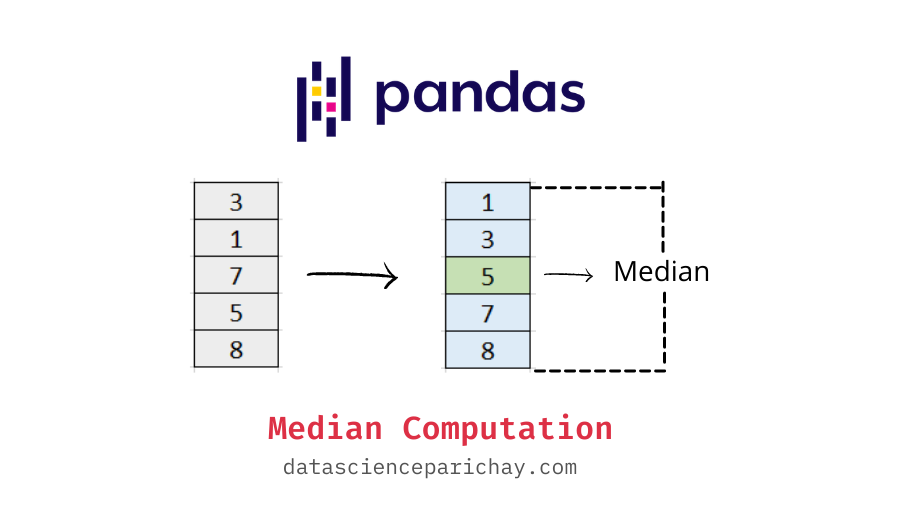# Pandas – Get Median of One or More Columns

The median of a set of numbers represents the middle value if the numbers are arranged in sorted order. It is a measure of central tendency and is often preferred over the mean as it’s not much affected by the presence of outliers. In this tutorial, we will look at how to get the median of one or more columns in a pandas dataframe.

You can use the pandas `median()` function or the pandas `quantile()` function to get the median of column values in a pandas dataframe. The following is the syntax:

```# median of single column
df['Col'].median()
# median of single column with quantile()
df['Col'].quantile(0.5)
# median of all numerical columns in dataframe
df.median()
# median of all numerical columns in dataframe with quantile()
df.quantile(0.5)```

Let’s create a sample dataframe that we will be using throughout this tutorial to demonstrate the usage of the methods and syntax mentioned.

```import pandas as pd

# create a dataframe
df = pd.DataFrame({
'sepal_legth': [5.1, 4.9, 4.7, 4.6, 5.0, 5.4, 4.6, 5.0],
'sepal_width': [3.5, 3.0, 3.2, 3.1, 3.6, 3.9, 3.4, 3.4],
'petal_length': [1.4, 1.4, 1.3, 1.5, 1.4, 1.7, 1.4, 1.5],
'petal_width': [0.2, 0.2, 0.2, 0.2, 0.2, 0.4, 0.3, 0.2],
'sepices': ['setosa']*8
})
# display the dataframe
print(df)```

Output:

```   sepal_legth  sepal_width  petal_length  petal_width sepices
0          5.1          3.5           1.4          0.2  setosa
1          4.9          3.0           1.4          0.2  setosa
2          4.7          3.2           1.3          0.2  setosa
3          4.6          3.1           1.5          0.2  setosa
4          5.0          3.6           1.4          0.2  setosa
5          5.4          3.9           1.7          0.4  setosa
6          4.6          3.4           1.4          0.3  setosa
7          5.0          3.4           1.5          0.2  setosa```

The sample dataframe is taken form a section of the Iris dataset. This sample has petal and sepal dimensions of eight data points of the “Setosa” species.

First, let’s see how to get the median of a single dataframe column.

You can use the pandas series `median()` function to get the median of individual columns (which essentially are pandas series). For example, let’s get the median of the “sepal_length” column in the above dataframe.

```# median of sepal_length column
print(df['sepal_length'].median())```

Output:

📚 Data Science Programs By Skill Level

Introductory

Intermediate ⭐⭐⭐

🔎 Find Data Science Programs 👨‍💻 111,889 already enrolled

Disclaimer: Data Science Parichay is reader supported. When you purchase a course through a link on this site, we may earn a small commission at no additional cost to you. Earned commissions help support this website and its team of writers.

`4.95`

You see that we get the median of all values in the “sepal_length” column as the scaler value 4.95.

Additionally, you can also use pandas `quantile()` function which gives the nth percentile value. Median is the 50th percentile value. So, to get the median with the `quantile()` function, pass 0.5 as the argument.

```# median of sepal_length column using quantile()
print(df['sepal_length'].quantile(0.5))```

Output:

`4.95`

Use the pandas dataframe `median()` function to get the median values for all the numerical columns in the dataframe. For example, let’s get the median of all the numerical columns in the dataframe “df”

```# mean of multiple columns
print(df.median())```

Output:

```sepal_length    4.95
sepal_width     3.40
petal_length    1.40
petal_width     0.20
dtype: float64```

We get the result as a pandas series.

Additionally, you can use the pandas dataframe `quantile()` function with an argument of 0.5 to get the median of all the numerical columns in a dataframe. Let’s use this function on the dataframe “df” created above.

```# mean of multiple columns using quantile()
print(df.quantile(0.5))```

Output:

```sepal_length    4.95
sepal_width     3.40
petal_length    1.40
petal_width     0.20
Name: 0.5, dtype: float64```

You can see that we get the median of all the numerical columns present in the dataframe.

Note that you can also use the pandas `describe()` function to look at key statistics including the median values of the numerical columns in the dataframe.

```# get dataframe statistics
df.describe()```

Output:

The median here is represented by the 50% value (that is, the value at the 50th percentile).

For more on the pandas dataframe median() function, refer to its documention.

With this, we come to the end of this tutorial. The code examples and results presented in this tutorial have been implemented in a Jupyter Notebook with a python (version 3.8.3) kernel having pandas version 1.0.5

•